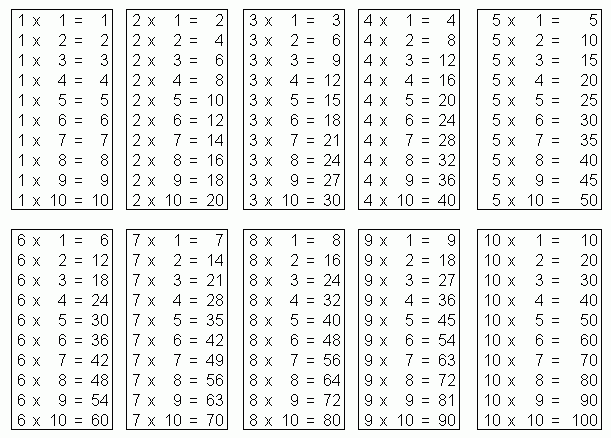Name: ___________________Date:___________________

 Email us to get an instant 20% discount on highly effective K-12 Math & English kwizNET Programs!

### Grade 4 - Mathematics3.9 Times Tables

 Times TableDirections: Review the following tables and answer the following questions. Also write at least ten examples of you own and times table for numbers 1 through 10 on a sheet of paper.
 Q 1: 5 x 6 =30352933 Q 2: 7 x 12 =968494108 Q 3: 11 x 11 =144110131121 Q 4: 10 x 12 =110115120100 Q 5: 9 x 11 =999590108 Q 6: 6 x 12 =84726282 Q 7: 8 x 9 =82726284 Q 8: 3 x 12 =46363315 Question 9: This question is available to subscribers only! Question 10: This question is available to subscribers only!